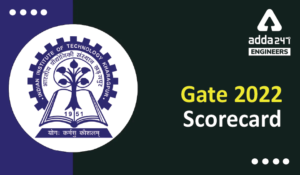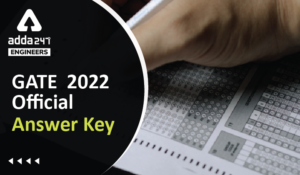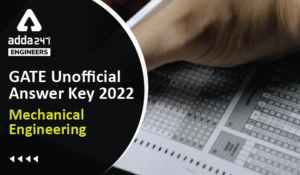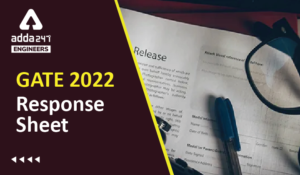Engineering Jobs   »   Electrical Engineering quizs   »   GATE 2022, GATE ELECTRICAL QUIZ,

# GATE’22 EE: Daily Practices Quiz 11-Dec-2021

## GATE Exam 2022

Gate 2022 Exam Date: IIT Kharagpur has been officially decided to take charge of conducting the GATE 2022 exams. The authorities have announced exam dates for GATE 2022 on the official website @gate.iitkgp.ac.in. The Candidates who have registered themselves for the GATE 2022 Exam can download their admit cards from 3rd January 2022 through the official website. The exam dates for GATE 2022 are 5th,6th,12th,13th February 2022. The Candidates can check their GATE 2022 Result from 17th March 2022 on the official website. In this article, we have covered important information regarding GATE Exam Date 2022.

## GATE’22 EE: Daily Practices Quiz

GATE’22 EE: Daily Practices Quiz 11-Dec-2021

Each question carries 2 marks
Negative marking: 2/3 mark
Total Questions: 10
Total marks: 20
Time: 20 min.

Q1. If a generator of 250 MVA has an inertia constant of 6 MJ/MVA, its inertia constant on a 100 MVA base is:
(a) 2.4 MJ/MVA
(b) 10.5 MJ/MVA
(c) 6 MJ/MVA
(d) 15 MJ/MVA

Q2. The Laplace transformation of I(t) is given by
I(s)=1/(s(s+4))
As t→ꝏ, the value of I(t) tends to….
(a) 0
(b) 4
(c) ꝏ
(d) 1/4

Q3. In a transistor when emitter current is increased from 6mA to 10 mA, the collector current increases 4mA to 7.80 mA. The value of β of the transistor is
(a) 9
(b) 10
(c) 19
(d) 49

Q4. Transistor is operated in its ___________ region when it works as an amplifier.
(a) Cut-off
(b) Saturation
(c) Active
(d) All of the above

Q5. When short -circuit test on a transformer is performed at 25V, 50 Hz, the drawn current I_1 is at a lagging p.f. angle of φ_1. If test is performed at 25 V, 25 Hz and drawn current I_2 is at a lagging p.f. angle of φ_2, then
(a) I2 > I1 and φ2 < φ1
(b) I2 < I1 and φ2 < φ1
(c) I2 > I1 and φ2 > φ1
(d) I2 < I1 and φ2 > φ1

Q6. A moving coil instrument having meter resistance of 5Ω is to be used as a voltmeter of range 0-100 V. If the full-scale deflection current is 10 mA, then required series resistance is………….
(a) 20Ω
(b) 1000Ω
(c) 9995Ω
(d) None of these

Q7. Megger is an instrument by which we can measure
(a) high resistance
(b) low resistance
(c) high current
(d) high voltage

Q8. Which is not the ideal characteristics of an op-amp?
(a) Output impedance = 0
(b) Bandwidth = ꝏ
(c) Open loop voltage gain =ꝏ
(d) Input resistance = 0

Q9. In case of a single-pulse width modulation with the pulse width = 2d, to eliminate the nth harmonic from the output voltage
(a) nd= π
(b) nd=2 π
(c) d= π
(d) 2d= π

Q10. Determine the rating of a transformer to deliver a 100 W of dc power to a load under full-wave bridge rectifier.
(a) 144.5 VA
(b) 123 VA
(c) 100 VA
(d) 150 VA

## SOLUTIONS

S1. Ans.(d)
Sol. ∵ H×S is constant.
∴Hnew Snew=Hold Sold⇒Hnew=(Hold Sold)/Snew =(6×250)/100=15 MJ/MVA

S2. Ans.(d)
Sol.
I(s)=1/(s(s+4))S3. Ans.(c)
Sol. Given,
∆IE=6 mA to 10 mA
∆IC=4mA to 7.80 mA
(∆IE=∆IC+∆IE )
∆IB = 2mA to 2.20 mA
β=(∆IC)/(∆IB )
=(7.80-4)/(2.20-2)=3.80/0.20
(β=19)

S4. Ans.(c)
Sol. A transistor can amplify a signal only when it is operated in active region.
Active region is also called a linear region. A transistor operates in active region when emitter base junction is forward biased and collector base junction is reverse biased.

S5. Ans.(c)
Sol. SC test of transformer: short circuit test gives the copper losses; these losses are taken into consideration by series parameters of the equivalent circuit. While, Open circuit test gives us iron losses; which are shown by parallel components of equivalent circuit.
Power factor(cos⁡ϕ )=R/Z=R/((R+j2πfL))⇒cos⁡〖ϕ α 1/f〗
And I=V/Z=V/((R+j2πfL)⇒I α 1/f
So, during SC test of transformer, both power factor and magnitude of current is inversely proportional to frequency(f).
Therefore, as frequency of a transformer decreases then both power factor and current increases and vice-versa.

S6. Ans.(c)
Sol. Series resistance,
Rs=V/Im -Rm=(100 V)/(10 mA)-5=10000-5=9995Ω

S7. Ans.(a)
Sol. The two instruments you will use most often to check continuity, or to measure the resistance of a circuit or circuit component, are the OHMMETER and the MEGGER (MEGOHMMETER). The ohmmeter is widely used to measure resistance and to check the continuity of electrical circuits and devices. Its range usually extends to only a few megohms. The megger is widely used for measuring insulation resistance, such as that between a wire and the outer surface of its insulation, and the insulation resistance of cables and insulators. The range of a megger can be extended to more than 1,000 megohms.

S8. Ans.(d)
Sol. Input impedance of an ideal op-amp= ꝏ

S9. Ans.(a)
Sol. To eliminate n^th harmonics, nd= π.

S10. Ans.(b)
Sol. Tranformer rating=Pdc/TUF
Where, TUF= transformer utilization factor
For, full wave bridge rectifier, TUF=0.812
For, centre-tapped, TUF=0.692
∴Transformer rating= 100/0.812=123 VA(case of bridge rectifier)

Sharing is caring!

Thank You, Your details have been submitted we will get back to you.

### TOPICS:

•HPU AE Recruitment Through GATE 2022, No...
•GATE 2022 Scorecard Released, Check Step...
•GATE Result 2022 OUT Live Check Your GAT...
•GATE 2022 Official Answer Key Out, Downl...
•GATE 2022 Answer Key Mechanical Engineer...
•GATE 2022 Response Sheet, Direct Link to...